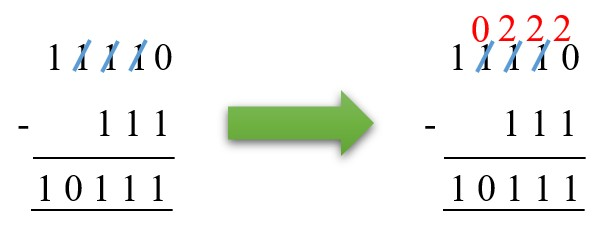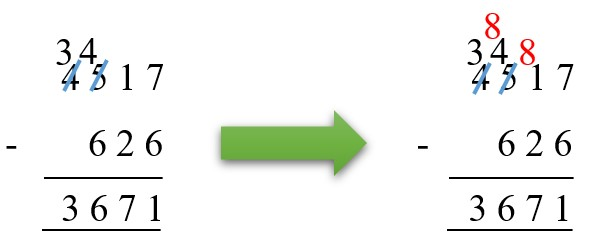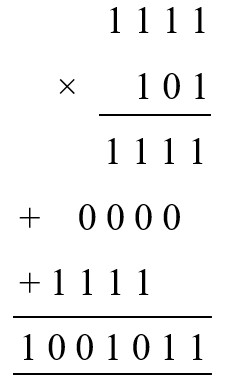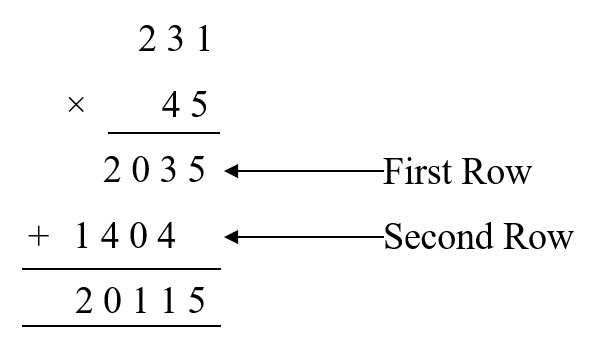4 Ijesha Close, Ilupeju, Lagos
+2348 097 685 118

#### Number Bases

• To convert a number from base ten to another base, use repeated division as follows:
• Divide the base ten number by the new base number,
• Continue dividing until zero is reached, writing down any remainders each time,
• Start at the last remainder and read upwards to get the new number.
• To convert a number from another base to base ten, either expand the given number or use repeated multiplication.
• To convert from one base to another base (that is not base ten), you would have to convert the first base to base ten, before you can convert it to the new base. For example, if you want to convert 34five to base eight, you would have to convert 34five to base ten first and then convert the base ten number to base eight.
• The digits that make up the number are always less than the base of the number. For example: In 123four, all the digits are less than the base.
• When adding, subtracting, multiplying, or dividing numbers in different bases, all the numbers must be lower than the base you are working with. Check out examples 5 and 6 below.
###### Examples
1. Convert 37ten to
1. Base eight
• 37 ÷ 8 = 4 remainder 5
• 37 = 4 eights + 5 units
• ∴ 37ten = 45eight
2. Base five
• Since 37 > 25, there must be a twenty-five in 37
• 37 ÷ 25 = 1 remainder 12
• 37 = 1 twenty-five + 12 units
• Consider the 12 units. Since 12 > 5, there must be some fives in 12
• 12 ÷ 5 = 2 remainder 2
• 12 = 2 fives + 2 units
• ∴ 37 = 1 twenty-five + 2 fives + 2 units
•         = 1 × 5² + 2 × 51 + 2 × 1
• ∴ 37ten = 122five
2. Convert 105ten to a binary number.
•• ∴ 105ten = 1101001two
3. Convert 100110two to
1. a base ten number
• 100110two = 1 × 25 + 0 × 24 + 0 × 23 + 1 × 22 + 1 × 21 + 0 × 20
•                    = 32 + 0 + 0 + 4 + 2 + 0
• ∴ 100110two = 38ten
2. a base eight number
• 38ten = 32 + 6
•           = 4 × 81 + 6 × 80
•           = 46eight
• ∴ 100110two = 46eight
4. Find the value of
1. (101two
• Firstly, convert the base two number to base ten: 101two = 1 × 2² + 0 × 21 + 1 × 20 = 4 + 0 + 1 = 5ten
• Next, find the cube of the number: (5ten)³ = 125ten
• Next, convert the number to base two:• ∴ (101two)³ = 1111101two
2. the square root of 100100two in base two
• 100100two = 1 × 25 + 0 × 24 + 0 × 23 + 1 × 22 + 0 × 21 + 0 × 20 = 32 + 4 = 36ten
• Square root of 36ten = 6ten
• 6ten to base two:• ∴ The square root of 100100two is 110two
5. Calculate the following:
1. 1101two + 1011two
•• Moving from right to left:
• The first column: 1 + 1 = 2, 2 is not less than the base 2. Divide 2 by 2 (the base), which would give 1 remainder 0, write down the remainder and carry 1.
• The second column: 1 + 0 + 1 = 2, the same rule applies here also; which would give 1 remainder 0, write down the remainder and carry 1.
• The third column: 1 + 1 + 0 = 2, divide by 2 and write down the remainder and carry 1.
• The fourth column: 1 + 1 + 1 = 3, divide by 2 and write down the remainder and carry 1.
• The fifth column: 1 + 0 = 1
2. 1101three + 1011three
•• Moving from right to left:
• The first column: 1 + 1 = 2. Write down 2 because it is less than the base we are working with.
• The second column: 0 + 1 = 1. Write down 1.
• The third column: 1 + 0 = 1. Write down 1.
• The fourth column: 1 + 1 = 2. Write down 2.
3. 344five + 43five
•• Moving from right to left:
• The first column: 4 + 3 = 8. Divide 7 by 5 (the base we are working with), which would give 1 remainder 2. Write down the remainder and carry the whole number 1 to the number on the left.
• The second column: 1 + 4 + 4 = 9. Divide 9 by 5, which would give 1 remainder 4. Write down the remainder and carry 1.
• The third column: 1 + 3 + 0 = 4. Write down 4 because it is less than 5 (the base we are working with).
4. 11110two – 111two
•• Moving from right to left:
• The first column: 0 is greater than 1. To make this subtraction possible, we have to borrow from the number to the left of it. In number bases, when we borrow, we borrow the base; in this case, 2. Therefore, (2 + 0) – 1 = 1.
• The second column: After borrowing 1, 0 is left, which is greater than 1. We would have to borrow again. Therefore, (2 + 0) – 1 = 1
• The third column: After borrowing, (2 + 0) – 1 = 1
• The fourth column: 0 – 0 = 0
• The fifth column: 1 – 0 = 0
5. 4517eight – 626eight
•• Moving from right to left:
• The first column: 7 – 6 = 1
• The second column: Borrow 1 from 5. Remember, when you borrow 1 in number bases, you are actually borrowing the base you are working with. Therefore, (8 + 1) – 2 = 7
• The third column: (8 + 4) – 6 = 6
• The fourth column: 3 – 0 = 3
6. Calculate the following:
1. 1111two × 101two
•2. 231six × 45six
•• For the first row (moving from right to left):
• The first column: 5 × 1 = 5 and 5 is less than 6 (the base we are working with), so, we write down 5.
• The second column: 5 × 3 = 15. 15 is greater than 6.  Divide 15 by 6, the result would be 2 remainder 3. Write down 3, carry 2.
• The third column: (5 × 2) + 2 (which was carried from the second column) = 12. 12 is greater than 6. Divide 12 by 6, the result would be 2 remainder 0. Write down 0, carry 2.
• The fourth column: (5 × 0) + 2 (carried from the third column) = 2. 2 is less than 6. Write down 2.
• For the first row (moving from right to left):
• The first column: 4 × 1 = 4. Write down 4.
• The second column: 4 × 3 = 12. 12 is greater than 6. Divide 12 by 6, the result would be 2 remainder 0. Write down 0, carry 2.
• The third column: (4 × 2) + 2 = 10. 10 is greater than 6. Divide 10 by 6, the result would be 1 remainder 4. Write down 4, carry 1.
• The fourth column: (4 × 0) + 1 = 1. Write down 1.
• Adding the first and second rows:
• The first column: 5 + 0 = 5. Write down 5.
• The second column: 3 + 4 = 7. Divide 7 by 6, the result would be 1 remainder 1. Write down 1 and carry 1.
• The third column: (0 + 0) + 1 = 1. Write down 1.
• The fourth column: 2 + 4 = 6. 6 is equal to 6. Remember, the numbers should be less than the base. Divide 6 by 6, the result would be 1 remainder 0. Write down 0, carry 1.
• The fifth column: 1 + 1 = 2. Write down 2.